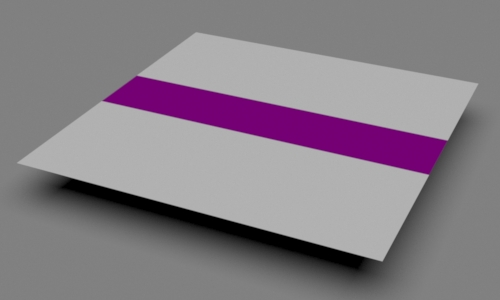### OSLRotating Texture Space

#### Introduction

There are occasions when a shader will not use 's' and 't' directly but will, instead, use rotated copies of them so that a texture map or a procedural pattern can be pivoted around a point defined in texture space - figures 1 and 2.Figure 1Figure 2 pivot point s = 0.5, t = 0.5

This tutorial shows how the built-in OSL function `rotate()` can be used for texture space rotations.

##### The rotate() Function

The function operates on 3d points.

 ```point outPnt = rotate(point inPnt, // 3d point to be rotated float angle, // angle of rotation, in radians point axis0, // 3d point defining one end of the rotational axis point axis1); // 3d point defining the other end of the rotational axis```

 To use the rotate() function the 's' and 't' texture coordinates must define two of the three coordinates of a 3d point - listing 1,

Listing 1 (rotateST.osl)

 ```shader rotateST(float s = 0 [[int lockgeom = 0]], float t = 0 [[int lockgeom = 0]], float angle = 0, float pivot_s = 0.5, float pivot_t = 0.5, output float resultS = 0, output float resultT = 0) { /* Use 's' and 't' to define the 'xz' coordinates of a 3d point */ point pntST = point(s, 0, t); /* The rotate function requires 2 points to define an axis of rotation - here represented by the 'y' axis. 'pivot_s' and 'pivot_t' are the coordinates of the center of rotation. */ point p1 = point(pivot_s, 0, pivot_t); point p2 = point(pivot_s, 1, pivot_t); point rotatedST = rotate(pntST, radians(angle), p1, p2); resultS = rotatedST; resultT = rotatedST; }```

##### Using rotateST in a Shading Network

A shader that creates a band of color is shown in listing 2. It has two modes of use. Either it creates a pattern using the 't' values it receives from a primvar. Or, if the 'T' parameter is connected it ignores the primvar and uses the values from the connection.Figure 3
Rollover images showing isconnected() not working and working.

In Rib the shading network might be:

```    Pattern "PxrOSL" "rotateST1" "string shader" "rotateST"
"float angle" 40
"float pivot_s" 0.5
"float pivot_t" 0.5
Pattern "PxrOSL" "band1" "string shader" "band"
"reference float T" ["rotateST1:resultT"]
"float t_position" 0.5
"float t_width" 0.2
"color patcolor" [0.4 0 0.4]
"color bakcolor" [1 1 1]
Bxdf "PxrDisney" "PxrDisney1"
"reference color baseColor" ["band1:resultRGB"]```

Switching from primvar 't' to parameter 'T' depends on detecting if 'T' is connected, hence, the use of the OSL function `isconnected()`. Unfortunately, the function is broken and will only become operational when RPS 21 is released.

Listing 2 (band.osl)

 ```shader band(float s = 0 [[int lockgeom = 0]], float t = 0 [[int lockgeom = 0]], float T = 0 [[string help = "ignored if not connected"]], float t_position = 0.5, float t_width = 0.2, color patcolor = color(0.4,0.0,0.4), color bakcolor = color(1,1,1), output color resultRGB = 0) { float upper = t_position - t_width/2; float lower = t_position + t_width/2; float tt = (isconnected(T)) ? T : t; if(tt >= upper && tt <= lower) resultRGB = patcolor; else resultRGB = bakcolor; } ```